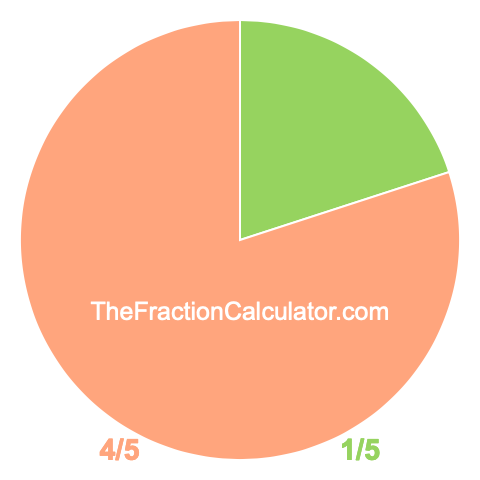Pie Chart of 1/5

To create a pie chart showing you how much 1/5 is, we first divided the pie (or circle) into 5 equal parts. Then we colored 1 of the 5 parts green. Here is our result:The pie chart of 1/5 not only gives you a visual of how much 1/5 of a whole is, but also shows you how much of the whole is not 1/5. To calculate what is not 1/5 of the pie chart, we simply subtracted 1/5 from one.

Pie Chart Fraction Maker
Get the pie chart for another fraction here. Make sure to submit a fraction where the numerator is smaller than the denominator.

/

Pie Chart of 1/6
Here is the next pie chart we have created for you.